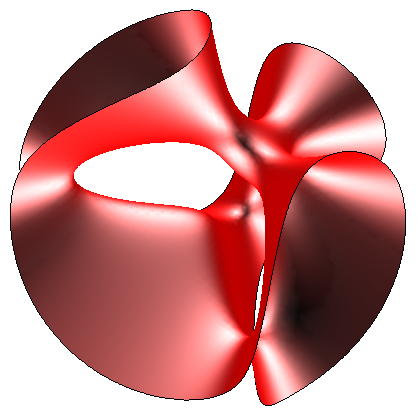# 27 lines on a cubic

Some of you may have noticed a particularly queer post on cp4space yesterday (since deleted), which was written by an impostor. Specifically, I was hosting several people (including, but by no means limited to, the daughter of Kjartan Poskitt). I happened to leave the room to acquire a bottle of Sandringham apple juice; in the interim, someone accessed my computer and left an entertaining message on cp4space. I apologise for any inconvenience or confusion caused by this complete and utter travesty.

Quite a while ago, I mentioned the hyperboloid of one sheet as an example of a doubly-ruled surface, where every point is determined by two straight lines contained by the surfaces. These are very rare. Much more common are singly-ruled surfaces, such as the cone, where we have a single straight line passing through each point on the surface in general position.

Non-singular cubics are very interesting beasts. In two dimensions, these are elliptic curves, which have a beautiful Abelian group structure with major applications in cryptography and number theory. If we add another dimension, then we obtain a cubic surface, such as this one:It transpires that on a non-singular cubic surface, there lie a total of 27 straight lines (when considered in complex projective 3-space). They are all realised as real lines in the Clebsch cubic, described by the equations$x_0 + x_1 + x_2 + x_3 + x_4 = 0$ and$x_0^3 + x_1^3 + x_2^3 + x_3^3 + x_4^3 = 0$. The first of these determines a three-dimensional subspace of projective 4-space; the second gives a cubic surface inhabiting that space. The cubic surface shown above is a visualisation of the Clebsch cubic in$\mathbb{R}^3$.

A particular Wolfram demonstration allows you to experiment with up to 24 lines on a cubic surface (the remaining three are at infinity). For certain values of the parameter, fewer lines are visible due to either singularities or the existence of non-real lines.

This entry was posted in Uncategorized. Bookmark the permalink.

### One Response to 27 lines on a cubic

1.pzq_alex says:

What about non-singular cubics in the complex projective 4-space? Are they 81-ruled or anything?